# How to add Formulas to Tables in Microsoft Word

Word 2013 tables look like cells in an Excel spreadsheet, but unlike Excel, you can’t start a formula just by typing an equal sign. Tables are a great thing to use because it provides a great way to provide structured ways to display information. You can easily add formulas tables to Microsoft Excel, but what about Microsoft Word, is it possible there?

Yes, it is. In this article, I am going to talk about how you can use formulas inside the Word table. There are a few formulas you can use, but it is enough to get a total, count, round number, etc. With the data on your table, it takes just a few clicks to add a formula. Also, if you are already familiar with Excel, Word formulas will be used as a piece of cake.

Contents

## How to add Tables to Microsoft Word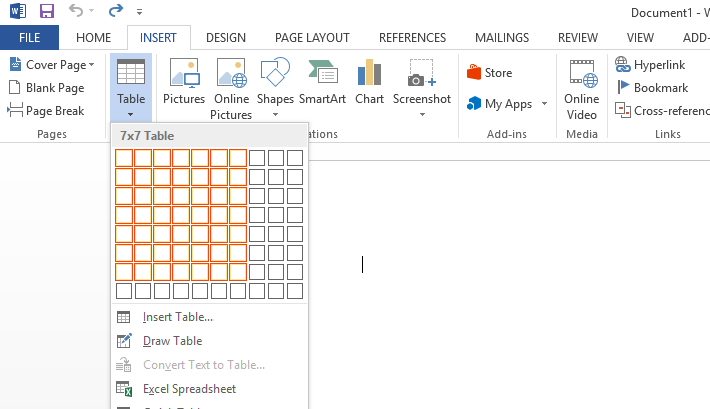Before adding formulas to a Table in Word, you must first add the Table to the Word document before everything else. It can be done via the Insert tab. You can create a calendar template using Microsoft Word. Once your table is inserted, go ahead and add some information. I have created a very simple table with a few numbers for my example.

## How to Insert Formula in Word Table

It is possible to discard one or more formulas in a Microsoft Word document. The following information will teach you how:

1. Layout tab and Formula
3. What about the Number Format?
4. Paste previously used Functions
5. Example Maximum Formula

## 1] Layout tab and Formula

OK, we should start by clicking on the Layout tab of the Microsoft Word Ribbon, and from there look for the Formula option and select it. The formula is located just to the right of the ribbon, so you can never miss it even if you try.

Click the target cell in your table, open the Layout tab and click Formula in the Data section. The next move you will want to make after selecting the Formula button is to add a formula. There are several ways to get this done, but one must follow a particular rule. You see, it is essential to include the equal sign.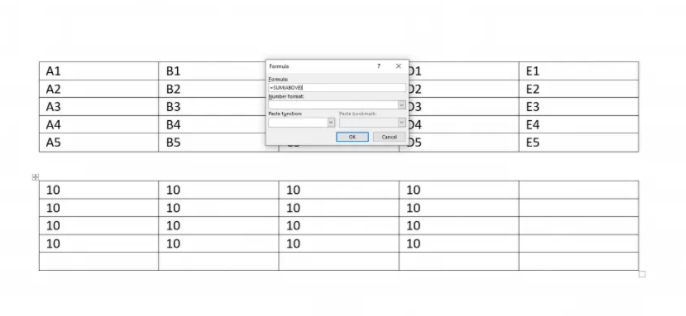For example, the formula must look like the following:

 =SUM(BELOW)

The word that comes after the equal sign is deemed as a function, and there are quite a few of them. As for the words in the bracket, they are the positions. Folks can use ABOVE, BELOW, LEFT, and RIGHT. It is also possible to combine positions, but it all depends on the location of the cells.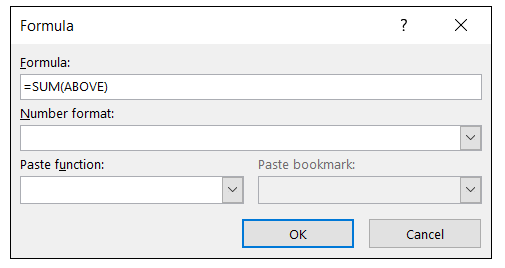Enter a formula using function = SUM to add cells. When you first open the Formula window, the word default formula = SUM (above). If you want to add all cells above the selected cell, you don’t need to change anything. To add cells from different directions, change the formula = SUM (BELOW), = SUM (LEFT) or = SUM (RIGHT).

## 3] What about the Number Format?

Let’s talk about formulas. Like Excel, a formula starts with an equal sign, then a function name, and an argument in parentheses. In Excel, you only specify cell references or named ranges such as A1: A3, etc. But in Word, you can use these positional terms.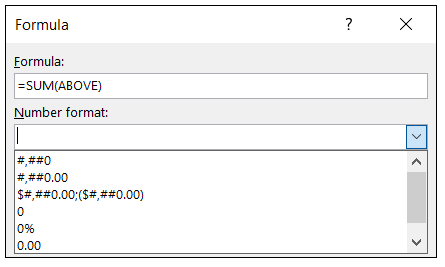You don’t have to add anything to the number format because it depends on what you want on your table. But if you are going, the options are a whole number, decimal, percentage, and currency. Choose a format for results like a coin, percentage, a whole number, or decimal. If you do not select a format, your results will match the cell format. Select a number format if necessary.

Without the formatting of the selected number, the formula results are displayed without the formatting, such as the “normal” formatting in Excel. Most of the time, you don’t have to use a format. Word lets you write your own layout using the same system as Excel. Click OK to finish the formula.

## 4] Paste previously used Functions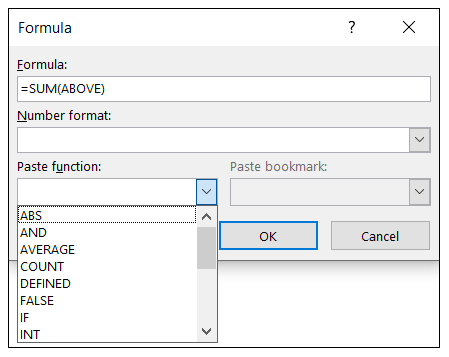If you want to get things done much faster than before, we recommend using the Paste Function feature. Here you will find previously used functions, so instead of going through the hassle of manually adding formulas every time, just use the paste function and that’s it. This puts the function in the Formula box, where you can simply add the location. You will find about twenty common functions in the list.

## 5] Example Maximum Formulas

In this first example, we want to see the maximum amount of our product. We enter MAX (above) and click “OK” in each cell where we want the number. Then, we see the largest amount for the cells above the formula.In this second example, we want the average cost and selling price instead of our product. We type AVERAGE (above) and click “OK” to see our results.

## Instructions on How to Insert Table Formulas in Word

1. To insert table formulas in Word, click into the table cell where you want to show the answer to the formula.
2. Then click the table’s “Layout” contextual tab in the Ribbon. In older versions of Word, this appears as the “Layout” tab of the “Table Tools” contextual tab in the Ribbon.
3. For all versions of Word, then click the “Formula” button in the “Data” button group to open the “Formula” dialog box.
4. If necessary, click into the “Formula:” field and enter the desired formula.
5. Optionally, to select a function to add to the formula shown in the “Formula:” field, use the “Paste function:” drop-down.
6. Optionally, to format the display of the numeric formula’s result, use the “Number format:” drop-down.
7. Then click the “OK” button to insert the formula field into the selected cell.

Overall, this is nothing close to Excel’s capabilities, but it is enough to compute some basic spreadsheets within Word. If you have any questions, feel free to comment. Enjoy!